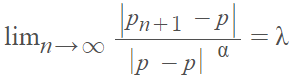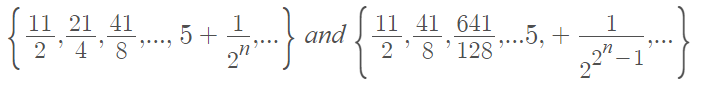# Asymptotic Error (Rate of Convergence)

Contents:

## What is the Asymptotic Error Constant?

The asymptotic error constant (λ) tells you something about the behavior of a sequence’s errors: differences between the terms of the sequence and the sequence’s limit. The asymptotic error constant affects the speed of convergence in conjunction with the order of convergence.

## How to Find the Asymptotic Error Constant

You can find a sequence’s asymptotic error constant λ with the following limit definition:Where:

• α = order of convergence,
• pn = a sequence,
• p = where the sequence converges (pn ≠ p).

As the number of terms increases, the sequence approaches the limit or horizontal asymptote; λ tells you the rate at which that happens. If positive constants λ and α exist for the above limit, then the sequence converges to p of order of α at rate λ.

In general, a sequence with a higher order of convergence will converge faster than a sequence with a lower order of convergence (the asymptotic constant also affects the speed of convergence, but not to the extent that the order does):

• If α = 1, pn converges to p linearly.
• If α = 2, pn converges to p quadratically.
• If α = 3, pn converges to p cubically.
• If 0 < α < 1, pn converges to p suberlinearly.
• If 1 < α < 2, pn converges to p superlinearly.

While there are many possible values for order of convergence, most of the sequences you’ll come across in beginning calculus and analysis classes will have α = 1 or α = 2.

For example, the following two sequences both converge to 5:However, they converge with different orders of convergence.

The first sequence converges linearly (α = 1) to 5:The second sequence converges quadratically (α = 2) to 5:## Asymptotic Error Constant: Example

Example question: What is the asymptotic error constant for the sequence:Step 1: Find the limit of the sequence:For this sequence, p = 0.

Step 2: Insert p from Step 1 and α from Step 2 into the formula and solve. Using the definition of the asymptotic error constant, the sequence has order of convergence α if λ exists in the limit and is finite. This particular sequence converges linearly (α = 1):## Order of ConvergenceThe order of convergence α affects how fast a sequence converges to its limit. It’s used in conjunction with the asymptotic error constant.

Larger values of α converge faster. For example:

• If 0 < α < 1, a sequence is suberlinearly convergent to p.
• If α = 1 (and λ < 1), a sequence is linearly convergent to p.
• If 1 < α < 2, a sequence is superlinearly convergent to p.
• If α = 2, a sequence is quadratically convergent to p.
• If α = 3, a sequence is cubically convergent to p.

If a sequence has a higher order of convergence, fewer iterations are needed to give a useful approximation.

Order of convergence looks at the relationship between successive error values. Basically, it measures the effectiveness of reducing the approximation error with each iteration. Big O notation is similar, but and gives the rate of convergence; It’s not sufficient to describe how fast the convergence is. For sequences that converge quickly, order of convergence is usually much better at describing speed of convergence .

## References

 Order of Convergence. Retrieved November 30, 2021 from: https://arnold.hosted.uark.edu/NA/Pages/OrderConv.pdf
Chapter 2: Solutions of Equations in One Variable. Retrieved August 14, 2021 from: https://www.math.tamu.edu/~smpun/MATH417/Chapter-2.pdf

CITE THIS AS:
Stephanie Glen. "Asymptotic Error (Rate of Convergence)" From StatisticsHowTo.com: Elementary Statistics for the rest of us! https://www.statisticshowto.com/asymptotic-error-rate-of-convergence/